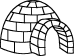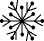EnchantedLearning.com is a user-supported site.
As a bonus, site members have access to a banner-ad-free version of the site, with print-friendly pages.

 You might also like: Divide Ten Pets Worksheet Printout Divide Six by 2, 3, and 6 Worksheet Printout Divide Nine Flowers Worksheet Printout Divide Eight Birds Worksheet Printout Divide Twelve Farm Animals Worksheet Printout Today's featured page: Numbers in French: A Book to Print

 Our subscribers' grade-level estimate for this page: 2nd - 3rd

 Go to the AnswersMath Pages EnchantedLearning.com Divide Fifteen Icy Items Divide the icy items into groups to do division. Visual Division PagesDivision Pages

 Divide the snowmen into three equal groups.How many snowmen are there? How many groups of snowmen are there? How many snowmen are in each group?
15 ÷ 3 =

 Divide the igloos into five equal groups.How many igloos are there? How many groups of igloos are there? How many igloos are in each group?
15 ÷ 5 =

 Divide the snowflakes into fifteen equal groups.How many snowflakes are there? How many groups of snowflakes are there? How many snowflakes are in each group?
15 ÷ 15 =

## Enchanted Learning Search

 Search the Enchanted Learning website for: